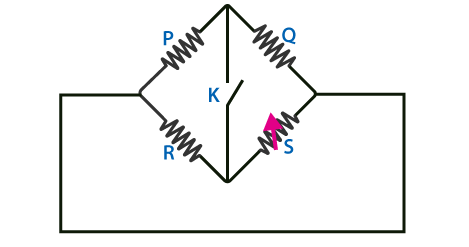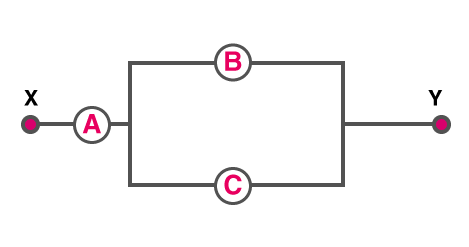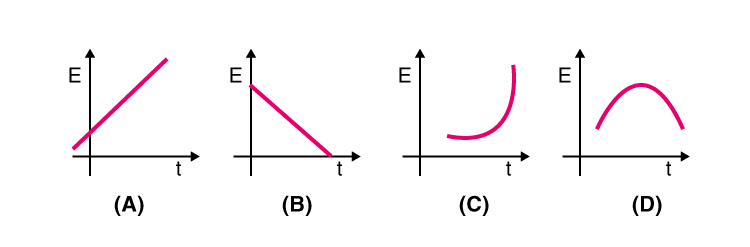## Physics MCQ on Current Electricity for NEET, JEE, Medical and Engineering Exam 2021

MCQ on Current Electricity: Previous trends reveal that NEET/JEE aspirants find Physics as the most challenging section amongst the other sections. But most of them fail to decipher the reason behind this. This particularly can be as the candidates taking up this examination are predominantly medical aspirants with their subject of interest being Biology. Also, sections like Physics are more on theories, laws, numerical as opposed to Biology which is more of fact-based, life sciences and comprising of substantial explanations.In this post we are providing you MCQ on Current Electricity, which will be beneficial for you in upcoming NEET, AIIMS, JEE, WBJEE, Medical and Engineering Exams.

## MCQ on Current Electricity

Q1. What is the principle behind thermocouple
a) Joule effect
b) Thomson effect
c) Seebeck effect
d) Peltier effect

(c) Seebeck effect

Q2. Select the graph which depicts the variation in thermoelectric power and the temperature difference between the hot and cold junction in the thermocouples(a)

Q3. Following is the Wheatstone bridge in which P/Q=R/S. Let K be the key which is closed, so what will be the deflection shown by galvanometer?a) The deflection happens in either side
b) There is no  deflation
c) Deflection happens on the right side
d) Deflection happens on the left side

(a) The deflection happens in either side

Q4. A potentiometer is considered a versatile and accurate device to make an electrical measurement of EMF. Why?
a) Because the method involves a combination
b) There are cells involved
d) As there is no flow of current through the galvanometer

(d) As there is no flow of current through the galvanometer

Q5. Consider three voltmeters A, B, and C with resistance R, 1.5R, and 3R respectively. Potential difference is applied across the points X and Y, the voltmeter readings are VA, VB, and VC.a) VA =VB ≠VC
b) VA VB ≠VC
c) VA = VB=VC
d) VA ≠ VB=VC

(c)VA = VB=VC

Q6. According to Faraday’s laws of electrolysis, to which of the following is the amount of decomposition proportional to?
a) The electrochemical equivalent of the substance
b) 1/current
c) 1/ electrochemicalequivalent
d) 1/timeforwhichcurrentpasses

(a) The electrochemical equivalent of the substance

Q7. What does the internal resistance of a cell mean?
a) The resistance of the material used in the cell
b) The vessel of the cell
c) The electrodes of the cell
d) The electrolyte used in the cell

(d) The electrolyte used in the cell

Q8. Following is the graph which represents the thermo EMF of two different metals which are joined end to end. One end of the metal is kept at a constant temperature while the other end is heated at very high temperature.
a)(d)

Q9. Consider copper and aluminium wire whose length and resistance are the same. Also, the specific resistance of aluminium is more than copper. Select the correct statement
a) Copper and aluminium mass are the same
b) Copper wire has no more mass than the aluminium wire
c) Copper wire has less mass than the aluminium wire
d) The information given is incomplete

(d) The information given is incomplete

Q10. Which of the following represents the relationship between the current flowing, i through the electric heater and the time taken, t.(b)

Q11. What is the ratio of power dissipated if two electric bulbs are connected in series and the ratio of their resistance is 1:2?

a) 1:4
b) 1:2
c) 2:1
d) 1:1

(b) 1:2